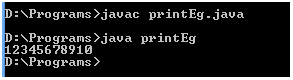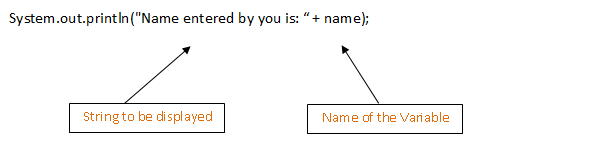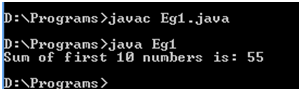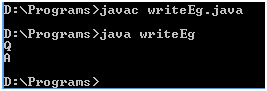# Writing Console Output in Java

Writing Console Output in Java refers to writing the output of a Java program to the console or any particular file.

The methods used for streaming output are defined in the PrintStream class. The methods used for writing console output are print(), println() and write().

## Methods of Writing Console Output in Java-print() and println()

Both print() and println() methods are used to direct the output to the console. These methods are defined in the PrintStream class and are widely used. Both these methods are used with the help of the System.out stream.

The basic differences between print() and println() methods are as follows:

• print() method displays the string in the same line whereas println() method outputs a newline character after its execution.
• print() method is used for directing output to console only whereas println() method is used for directing output to not only console but other sources also.

Now, let us understand it in a better way with the help of some examples.

Example of print() method:

1. // Sample program to display numbers from 1-10 using print method
2. class printEg
3. {
4.             public static void main(String args[])
5.             {
6.                         int a;
7.                         for (a=1 ;  a<=10 ;  a++)
8.                         {
9.                                     System.out.print(a);
10.                         }
11.             }
12. }

Output:Example of println() method:

Let us take the same example above and use println() method in place of print() method.

1. // Sample program to display numbers from 1-10 using println method
2. class printlnEg
3. {
4.             public static void main(String args[])
5.             {
6.                         int a;
7.                         for (a=1 ; a<=10 ; a++)
8.                         {
9.                                   System.out.println(a);
10.                         }
11.             }
12. }

Output:Observe the difference in the output.

Both these functions work best for output of strings as well. Let us have a look at another example.

### Displaying strings using print() and println() methods

It is very easy to display strings using print() and println() methods. For displaying any particular string, the string to be displayed on screen is written within the double quotation marks (i.e. “  “).

For example:     System.out.println(“Hello!! How are you?”);

In addition, if you want to display the value of any particular variable used in the program, then you have to append the variable name along with the string with a plus (+) symbol.Example for displaying any string output:

1. // Sample program to display sum of numbers from 1-10
2. class Eg1
3. {
4.             public static void main(String args[])
5.             {
6.                         int a, total = 0;
7.                         for (a=1 ; a<=10 ; a++)
8.                         {
9.                                     total = total + a;
10.                         }
11.                         System.out.println("Sum of first 10 numbers is: " + total);
12.             }
13. }

Output:## The write() method

Alternatively, you can make use of the write() method for directing the output of your program to the console. The easiest syntax of the write() method is:

void write(int b);

Where b is an integer of low order eight bits.

Let us have a look at a simple example.

1. class writeEg
2. {
3.             public static void main(String args[])
4.             {
5.                         int a, b;
6.                         a = 'Q';
7.                         b = 65;
8.                         System.out.write(a);
9.                       System.out.write('\n');
10.                        System.out.write(b);
11.                         System.out.write('\n');
12.             }
13. }

Output: